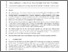Dhimish, Mahmoud, Holmes, Violeta, Mehrdadi, Bruce, Dales, Mark and Mather, Peter (2017) Photovoltaic fault detection algorithm based on theoretical curves modelling and fuzzy classification system. Energy, 140 (1). pp. 276-290. ISSN 0360-5442
Abstract

This work proposes a fault detection algorithm based on the analysis of the theoretical curves which describe the behavior of an existing PV system. For a given set of working conditions, solar irradiance and PV modules' temperature, a number of attributes such as voltage ratio (VR) and power ratio (PR) are simulated using virtual instrumentation (VI) LabVIEW software. Furthermore, a third order polynomial function is used to generate two detection limits for the VR and PR ratios obtained using VI LabVIEW simulation tool.

The high and low detection limits are compared with measured data taken from 1.1 kWp PV system installed at the University of Huddersfield, United Kingdom. Samples lie out of the detection limits are processed by a fuzzy logic classification system which consists of two inputs and one output membership function.

In this paper, PV faults corresponds to a short circuited PV module. The obtained results show that the fault detection algorithm can accurately detect different faults occurring in the PV system, where the maximum detection accuracy of before considering the fuzzy logic system is equal to 95.27%. However, the fault detection accuracy is increased up to a minimum value of 98.8% after considering the fuzzy system.

Information
Library
DocumentsPreview
Photovoltaic fault detection algorithm based on theoretical curves modelling and fuzzy classification system.pdf - Accepted Version